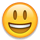# sum hours assigned to a contact on a sheet for a particular month

Example: I have a project that lasts three months on a project sheet.

I am required by the client to report out how many hours of work have been completed by each person assigned to a task at the end of each month on a dashboard

So for example: I need a formula that is going to Find all columns assigned to the contact "Timothy Lawrence" in August (Finish date column) and total those hours

Here is where I am getting stuck...

=SUMIF(Resource:Resource, FIND("Timothy Lawrence", @cell) > 0, [Actual Hours]:[Actual Hours])

This finds all of the hours worked by Timothy Lawrence on the sheet, however I can't seem to figure out how to narrow it down by the month.

The way I logic it out in my head is: "Sumif resource is Timothy Lawrence and finish date is month 8"

I have tried the formula below but get an Invalid data type:

=SUMIF(MONTH(8), AND(Resource:Resource, FIND("Timothy Lawrence", @cell) > 0, [Actual Hours]:[Actual Hours]))

Any thoughts? I'm stuck using the sheet template provided by the client (just adding formula rows to the bottom) so an actual re-write of the sheet isn't possible.

• This is where SUMIFS comes in handy since you're summing under more than one condition.

Try =SUMIFS([your actual hours range],[range of column with the names],"Timothy Lawrence",[range of column with the months],8)

And don't forget in all these ranges to put \$ signs, so as you drag the SUMIFS formula down, it doesn't adjust the numbers. So it may look like this:

=SUMIFS([Actual Hours]\$1:[Actual Hours]\$200,[Name]\$1:[Name]\$200,"Timothy Lawrence",[Month]\$1:[Month]\$200,8)

The first item in the parenthesis is the range of what you want to add, followed by ranges and criteria for any conditions.

• =SUMIFS([Actual Hours]:[Actual Hours], Resource:Resource, FIND("Timothy Lawrence", @cell) > 0, [Finish Date]:[Finish Date], MONTH(@cell) = ##)

Using a SUMIFS means you move the range to be summed to the first section (underlined). To account for the month, you would use your finish date column as the range and then use the MONTH(@cell) = ## as your criteria, replacing the ## with the appropriate month number.

If it is possible to have multiple years recorded on the same sheet, you can account for this by incorporating an AND function to add in the YEAR requirement.

=SUMIFS([Actual Hours]:[Actual Hours], Resource:Resource, FIND("Timothy Lawrence", @cell) > 0, [Finish Date]:[Finish Date], AND(MONTH(@cell) = ##, YEAR(@cell) = ####))

.

If you are referencing entire columns as indicated in your original formula, you do not need to use the \$ to lock in references unless you are planning to dragfill horizontally. Using the \$ to lock references when vertically dragfilling is only necessary if you are referencing specific row numbers which you are not.

• Thank you for taking the time to reply, this worked for me• Thank you so much for taking the time to reply, this worked for me!

I had to wrap the month in an IFERROR formula because for one of the people it kept throwing up an "invalid data type" for some reason, honestly not sure why it works fine with every other person on the sheet.

This is the formula that gives me the INVALID DATA TYPE error:

=SUMIFS([Actual Hours]:[Actual Hours], Resource:Resource, FIND("Bill Corrigan", @cell) > 0, Finish:Finish, (MONTH(@cell), 0) = 8)

However the formula below gives me the correct number of hours (43):

=SUMIFS([Actual Hours]:[Actual Hours], Resource:Resource, FIND("Bill Corrigan", @cell) > 0, Finish:Finish, IFERROR(MONTH(@cell), 0) = 8)

You brought up a good point of needed to reference years as well (if a project starts in December and ends in February for example.

What is the proper way of wrapping an AND statement with an IFERROR?

I am trying to use:

=SUMIFS([Actual Hours]:[Actual Hours], Resource:Resource, FIND("Bill Corrigan", @cell) > 0, Finish:Finish, IFERROR(AND(MONTH(@cell) = 8, YEAR(@cell) = 2019), 0))

But I'm still getting the INVALID DATA TYPE error.

• The IFERROR will typically be needed because of any blanks within the date range. I generally work that in to my own formulas. I am not sure why I didn't this time. Sorry about that.

The IFERROR function wouldn't be working on the AND function. It would be working on the MONTH and YEAR functions individually, so you would need to wrap them (not the AND) in the IFERROR.

=SUMIFS([Actual Hours]:[Actual Hours], Resource:Resource, FIND("Bill Corrigan", @cell) > 0, Finish:Finish, AND(IFERROR(MONTH(@cell), 0) = 8, IFERROR(YEAR(@cell), 0) = 2019))

• Thank you for the prompt reply and clarification!

• Sure thing. Happy to help!## Help Article Resources

Want to practice working with formulas directly in Smartsheet?

Check out the Formula Handbook template!